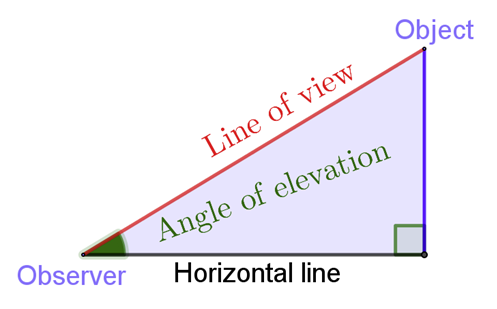Explore

# Height and distance

This resource demonstrates the meaning of angles of elevation and depression. There are examples and annotated questions for students to practise using trigonometry.

Year level(s) Year 10
Audience Teacher
Teaching strategies and pedagogical approaches Explicit teaching
Keywords Angle, Length, Height, Tangent, Cosine, Sine, Trigonometry, Depression, Elevation

## Curriculum alignment

Curriculum connections Critical and creative thinking
Strand and focus Space
Topics Pythagoras and trigonometry
AC: Mathematics (V9.0) content descriptions
AC9M10M03

Solve practical problems applying Pythagoras' theorem and trigonometry of right-angled triangles, including problems involving direction and angles of elevation and depression

Numeracy progression Understanding geometric properties (P7)
Understanding units of measurement (P10)